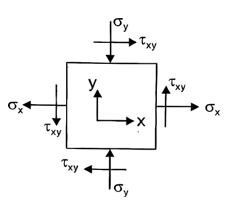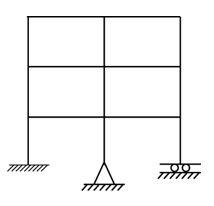# GATE’22 CE:- Daily Practice Quiz 14-July-2021

Quiz: Civil Engineering
Exam: GATE
Topic: Miscellaneous

Each question carries 2 mark
Negative marking: 1/3 mark
Time: 12 Minutes

Q1. The state of stresses on an element is shown in the given figure. The values of stresses are σ_x (=32MPa):σ_y (=-10MPa) and major principal stress σ_1 (=40 MPa). The minor principal stress ‘σ_2’ is(a) -22 MPa
(b) -18 MPa
(c) 22 MPa
(d) Indeterminable due to insufficient data

Q2. What is the limiting principle tensile stress in prestress uncracked concrete member of M25 grade?
(a) 1.2 MPa
(b) 1.5 MPa
(c) 2 MPa
(d) 2.5 MPa

Q3. A steady, two-dimensional, incompressible flow field is represented by u = x + 3y + 3 and V = 2x – y – 8 in this flow field, the stagnation point is
(a) (3, 2)
(b) (-3, 2.5)
(c) (-3, -2)
(d) (3, -2)

Q4. Which one of the following represents relative density of saturated sand deposited having moisture content of 25%, if maximum and minimum void ratio of sand are 0.95 and 0.45 respectively and specific gravity of sand particles is 2.6?
(a) 40%
(b) 50%
(c) 60%
(d) 70%

Q5. The kinematic indeterminacy of the structure shown in the figure is equal to(a) 14
(b) 15
(c) 16
(d) 17

Solutions

S1. Ans.(b)
Sol. σ_x+σ_y=σ_1+σ_2
32+(-10)=40+σ_2
▭(σ_2= -18 MPa)

S2. Ans.(a)
Sol. As per IS
Maximum principal tensile stress in prestressed concrete member = 0.24 √fck
=0.24√25
=0.24×5
=1.20 MPa

S3. Ans.(d)
Sol. Given,
u = x + 3y + 3
v = 2x – y – 8
For stagnation point u = 0 & v = 0
x+3y+3=0—-(1)
2x-y-8=0—-(2)
By solving equation (1) & (2)
▭(■(x=3@y= -2))⇒(3,-2)

S4. Ans.(c)
Sol. Given, S = 1 (Saturated sand)
e_max=0.95
e_min=0.45
w=25%=0.25
G=2.6
I_D=?
We know,
Se=WG
1×e=0.25×2.6
▭(e=0.65)
Now,
I_D=(e_max-e)/(e_max-e_min )
■(0.95-0.65 @0.95-0.45)×100
▭(I_D=60%)

S5. Ans.(a)
Sol. No.of inextensible members (m) =15
No.of external reactions (r_e) =7
No.of joints (j) =12
Internal hinged reactions (r_r) =0
Kinematic indeterminacy (D_k) =3j-r_e-m+r_r
=(3×12)-7-15+0
=36-22
▭(D_k=14)

×Join India's largest learning destination

What You Will get ?

•Job Alerts
•Daily Quizzes
•Subject-Wise Quizzes
•Current Affairs
•Previous year question papers
•Doubt Solving session

ORJoin India's largest learning destination

What You Will get ?

•Job Alerts
•Daily Quizzes
•Subject-Wise Quizzes
•Current Affairs
•Previous year question papers
•Doubt Solving session

ORJoin India's largest learning destination

What You Will get ?

•Job Alerts
•Daily Quizzes
•Subject-Wise Quizzes
•Current Affairs
•Previous year question papers
•Doubt Solving session

Enter the email address associated with your account, and we'll email you an OTP to verify it's you.Join India's largest learning destination

What You Will get ?

•Job Alerts
•Daily Quizzes
•Subject-Wise Quizzes
•Current Affairs
•Previous year question papers
•Doubt Solving session

Enter OTP

Please enter the OTP sent to
/6

Did not recive OTP?

Resend in 60sJoin India's largest learning destination

What You Will get ?

•Job Alerts
•Daily Quizzes
•Subject-Wise Quizzes
•Current Affairs
•Previous year question papers
•Doubt Solving sessionJoin India's largest learning destination

What You Will get ?

•Job Alerts
•Daily Quizzes
•Subject-Wise Quizzes
•Current Affairs
•Previous year question papers
•Doubt Solving session

Almost there

+91Join India's largest learning destination

What You Will get ?

•Job Alerts
•Daily Quizzes
•Subject-Wise Quizzes
•Current Affairs
•Previous year question papers
•Doubt Solving session

Enter OTP

Please enter the OTP sent to Edit Number

Did not recive OTP?

Resend 60

By skipping this step you will not recieve any free content avalaible on adda247, also you will miss onto notification and job alerts

Are you sure you want to skip this step?

By skipping this step you will not recieve any free content avalaible on adda247, also you will miss onto notification and job alerts

Are you sure you want to skip this step?